Home | | Modern Analytical Chemistry | LiquidÔÇôLiquid Extractions

# LiquidÔÇôLiquid Extractions

1. Partition Coefficients and Distribution Ratios 2. LiquidÔÇôLiquid Extraction with No Secondary Reactions 3. LiquidÔÇôLiquid Extractions Involving AcidÔÇôBase Equilibria 4. LiquidÔÇôLiquid Extractions Involving Metal Chelators

LiquidÔÇôLiquid Extractions

A liquidÔÇôliquid extraction is one of the most important separation techniques used in environmental, clinical, and industrial laboratories. Two examples from envi- ronmental analysis serve to illustrate its importance. Public drinking water supplies are routinely monitored for trihalomethanes (CHCl3, CHBrCl2, CHBr2Cl, and CHBr3) because of their known or suspected carcinogeneity. Before their analysis by gas chromatography, trihalomethanes are separated from their aqueous matrix by a liquidÔÇôliquid extraction using pentane.21 A liquidÔÇôliquid extraction is also used in screening orange juice for the presence of organophosphorous pesticides. A sample of orange juice is mixed with acetonitrite and filtered. Any organophospho- rous pesticides that might be present in the filtrate are extracted with petroleum ether before a gas chromatographic analysis.22

In a simple liquidÔÇôliquid extraction the solute is partitioned between two im- miscible phases. In most cases one of the phases is aqueous, and the other phase is an organic solvent such as diethyl ether or chloroform. Because the phases are im- miscible, they form two layers, with the denser phase on the bottom. The solute is initially present in one phase, but after extraction it is present in both phases. The efficiency of a liquidÔÇôliquid extraction is determined by the equilibrium constant for the soluteÔÇÖs partitioning between the two phases. Extraction efficiency is also in- fluenced by any secondary reactions involving the solute. Examples of secondary re- actions include acidÔÇôbase and complexation equilibria.

## Partition Coefficients and Distribution Ratios

Earlier we learned that the partitioning of a solute between two phases is described by a partition coefficient. If the solute is initially in an aqueous phase and is ex- tracted into an organic phase*A large value for KD indicates that the extraction of the solute into the organic phase is favorable.

In evaluating the efficiency of an extraction, however, we must consider the soluteÔÇÖs total concentration in each phase. We define the distribution ratio, D, to be the ratio of the soluteÔÇÖs total concentration in each phase.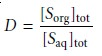When the solute exists in only one form in each phase, then the partition coefficient and the distribution ratio are identical. If, however, the solute exists in more than one form in either phase, then KD and D usually have different values. For example, if the solute exists in two forms in the aqueous phase, A and B, only one of which, A, partitions itself between the two phases, thenThis distinction between KD and D is important. The partition coefficient is an equilibrium constant and has a fixed value for the soluteÔÇÖs partitioning between the two phases. The value of the distribution ratio, however, changes with solution con- ditions if the relative amounts of forms A and B change. If we know the equilibrium reactions taking place within each phase and between the phases, we can derive an algebraic relationship between KD and D.

## LiquidÔÇôLiquid Extraction with No Secondary Reactions

In the simplest form of liquidÔÇôliquid extraction, the only reaction affecting extrac- tion efficiency, is the partitioning of the solute between the two phases (Figure 7.20). In this case the distribution ratio and the partition coefficient are equal.7.19Conservation of mass requires that the moles of solute initially present in one phase equal the combined moles of solute in the aqueous and organic phases after the extraction; thus7.20

where the subscript indicates the extraction number. The concentration of S in the aqueous phase after the extraction is7.21

whereas the soluteÔÇÖs concentration in the organic phase is7.22

where Vaq and Vorg are the volumes of the aqueous and organic phases. Solving equation 7.20 for (moles org)1 and substituting into equation 7.22 leave us with7.23

Substituting equations 7.21 and 7.23 into equation 7.19, we obtainRearranging and solving for the fraction of solute remaining in the aqueous phase after one extraction, (qaq)1, gives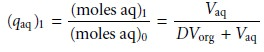7.24

The fraction present in the organic phase after one extraction, (qorg)1, isThe fraction present in the organic phase after one extraction, (qorg)1, is

Example 7.14 shows how equation 7.24 is used to calculate the efficiency of a simple liquidÔÇôliquid extraction.In Example 7.14 a single extraction results in an extraction efficiency of only 60%. If a second extraction is carried out, the fraction of solute remaining in the aqueous phase, (qaq)2, is given byIf the volumes of the aqueous and organic layers are the same for both extractions, then the cumulative fraction of solute remaining in the aqueous layer after two ex- tractions, (Qaq)2, isIn general, for a series of n identical extractions, the fraction of analyte remaining in the aqueous phase after the last extraction is7.25An important observation from Examples 7.14 and 7.15 is that an extraction efficiency of 99.9% can be obtained with less solvent when using multiple extrac- tions. Obtaining this extraction efficiency with one extraction requires 9990 mL of the organic solvent. Eight extractions using separate 15-mL portions of the or- ganic solvent, however, requires only 120 mL. Although extraction efficiency in- creases dramatically with the first few multiple extractions, the effect quickly di- minishes as the number of extractions is increased (Figure 7.21). In most cases there is little gain in extraction efficiency after five or six extractions. In Example 7.15 five extractions are needed to reach an extraction efficiency of 99%, and an additional three extractions are required to obtain the extra 0.9% increase in ex- traction efficiency.## LiquidÔÇôLiquid Extractions Involving AcidÔÇôBase Equilibria

In a simple liquidÔÇôliquid extraction the distribution ratio and the partition coeffi- cient are identical. As a result, the distribution ratio is unaffected by any change in the composition of the aqueous or organic phase. If the solute also partici- pates in a single-phase equilibrium reaction, then the distribution ratio and the partition coefficient may not be the same. For example, Figure 7.22 shows the equilibria occurring when extracting an aqueous solution contain- ing a molecular weak acid, HA, with an organic phase in which ionic species are not soluble. In this case the partition coefficient and the distribution ratio are7.267.27Since the position of an acidÔÇôbase equilibrium depends on the pH, the distribution ratio must also be pH-dependent. To derive an equation for D showing this depen- dency, we begin with the acid dissociation constant for HA.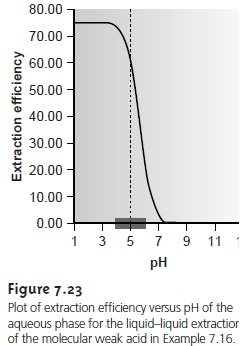The same approach can be used to derive an equation for the distri- bution ratio when the solute is a molecular weak base, B, (Figure 7.24). The resulting distribution ratio iswhere Ka is the acid dissociation constant for the weak baseÔÇÖs conjugate weak acid.

## LiquidÔÇôLiquid Extractions Involving Metal Chelators

One of the most common applications of a liquidÔÇôliquid extraction is the selective extraction of metal ions using a chelating agent. Unfor- tunately, many chelating agents have a limited solubility in water or are subject to hydrolysis or air oxidation in aqueous solutions. For these reasons the chelating agent is added to the organic solvent in- stead of the aqueous phase. The chelating agent is extracted into the aqueous phase, where it reacts to form a stable metalÔÇôligand complex with the metal ion. The metalÔÇôligand complex is then extracted into the organic phase. A summary of the relevant equilibria is shown in Figure 7.25.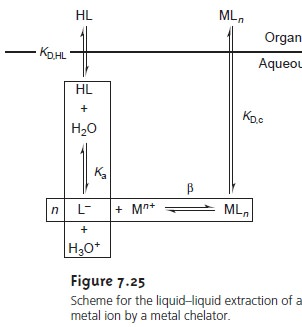If the ligandÔÇÖs concentration is much greater than the metal ionÔÇÖs concentration, the distribution ratio is given as*7.30

where CL is the initial concentration of ligand in the organic phase be- fore the extraction. The distribution ratio calculated using equation 7.30 can be substituted back into equation 7.25 to determine the extraction efficiency. As shown in Example 7.17, the extraction efficiency for metal ions shows a marked pH dependency.One of the advantages of using a chelating agent is the high degree of selec- tivity that it brings to the extraction of metal ions. As shown in Example 7.17 and Figure 7.26, the extraction efficiency for a divalent cation increases from approximately 0%ÔÇô100% over a range of only 2 pH units. Furthermore, a chelating agentÔÇÖs metalÔÇôligand formation constant varies substantially between metal ions. As a result, significant differences arise in the pH range over which different metal ions experience an increase in extraction efficiency from 0% to 100% (Figure 7.27).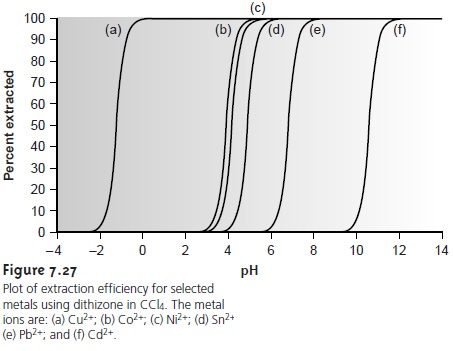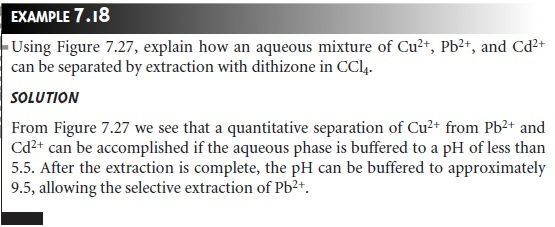LiquidÔÇôliquid extractions using ammonium pyrrolidine dithiocarbamate (APDC) as a metal chelating agent are commonly encountered in the analysis of metal ions in aqueous samples. The sample and APDC are mixed together, and the resulting metalÔÇôligand complexes are extracted into methyl isobutyl ketone before analysis.

Study Material, Lecturing Notes, Assignment, Reference, Wiki description explanation, brief detail
Modern Analytical Chemistry: Obtaining and Preparing Samples for Analysis : LiquidÔÇôLiquid Extractions |